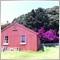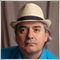# How to count how many times a conditional loop has been loop through?116

I am currently experimenting conditional loops and came across a problem which I need some help solving it. Please see below my codes.

Does MQL4 has a built in function called COUNT ?  So I can do something like count(i), which returns the amount of integers that passes the condition.

```// get a random number from 1-10
int num = 1 + 10*MathRand()/32768;
Print ("random= ",num);

// conditional loop, print out the numbers, Start from a random number(1~10) to 10
for(int i=1;i<=10;i++ ) {
if(i>num){
Print ("i= ",i);
}}

// Does MQL4 has a built in function called COUNT ?  So I can do something like count(i), which returns the amount of integers that passes the condition.
// eg: if num=8, Print(i) = 9, 10 ; count(i) = 2 because both 9 and 10 passes the condition. ```5068

Just increment a counter variable (standard coding practice).

```int MyCount = 0;
for( int i = 1; i <= 10; i++ )
{
if( i > num )
{
MyCount++;
Print( "i: ", i, "; Count: ", MyCount );
}
}```